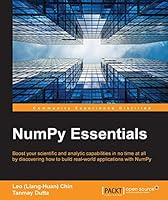# NumPy Essentials## Book Description

### Key Features

• Optimize your Python scripts with powerful NumPy modules
• Explore the vast opportunities to build outstanding scientific/ analytical modules by yourself
• Packed with rich examples to help you master NumPy arrays and universal functions

### Book Description

In today's world of science and , it's all about speed and flexibility. When it comes to scientific computing, NumPy tops the list. NumPy gives you both the speed and high productivity you need.

This book will walk you through NumPy using clear, step-by-step examples and just the right amount of . We will guide you through wider applications of NumPy in scientific computing and will then focus on the fundamentals of NumPy, including array objects, functions, and matrices, each of them explained with practical examples.

You will then learn about different NumPy modules while performing mathematical operations such as calculating the Fourier Transform; solving linear systems of equations, interpolation, extrapolation, regression, and curve fitting; and evaluating integrals and derivatives. We will also introduce you to using Cython with NumPy arrays and writing extension modules for NumPy code using the C API. This book will give you exposure to the vast NumPy library and help you build efficient, high-speed programs using a wide range of mathematical features.

### What you will learn

• Manipulate the key attributes and universal functions of NumPy
• Utilize matrix and mathematical computation using linear algebra modules
• Implement regression and curve fitting for
• Perform time frequency / spectral density analysis using the Fourier Transform modules
• Collate with the distutils and setuptools modules used by other Python libraries
• Establish Cython with NumPy arrays
• Write extension modules for NumPy code using the C API
• Build sophisticated data structures using NumPy array with libraries such as Panda and Scikits

Leo (Liang-Huan) Chin is a data engineer with more than 5 years of experience in the field of Python. He works for Gogoro smart scooter, Taiwan, where his job entails discovering new and interesting biking patterns . His previous work experience includes ESRI, California, USA, which focused on spatial-temporal data mining. He loves data, analytics, and the stories behind data and analytics. He received an MA degree of GIS in geography from State University of New York, Buffalo. When Leo isn't glued to a computer screen, he spends time on photography, traveling, and exploring some awesome restaurants across the world. You can reach Leo at http://chinleock.github.io/portfolio/.

Tanmay Dutta is a seasoned programmer with expertise in programming languages such as Python, Erlang, C++, Haskell, and F#. He has extensive experience in developing numerical libraries and frameworks for investment banking businesses. He was also instrumental in the design and development of a in Python (pandas, NumPy, and Django) for a wealth fund in Singapore. Tanmay has a master's degree in financial engineering from Nanyang Technological University, Singapore, and a certification in computational finance from Tepper Business School, Carnegie Mellon University.

Chapter 1. An Introduction To Numpy
Chapter 2. The Numpy Ndarray Object
Chapter 3. Using Numpy Arrays
Chapter 4. Numpy Core And Libs Submodules
Chapter 5. Linear Algebra In Numpy
Chapter 6. In Numpy
Chapter 7. Building And Distributing Numpy Code
Chapter 8. Speeding Up Numpy With Cython
Chapter 9. Introduction To The Numpy C-Api